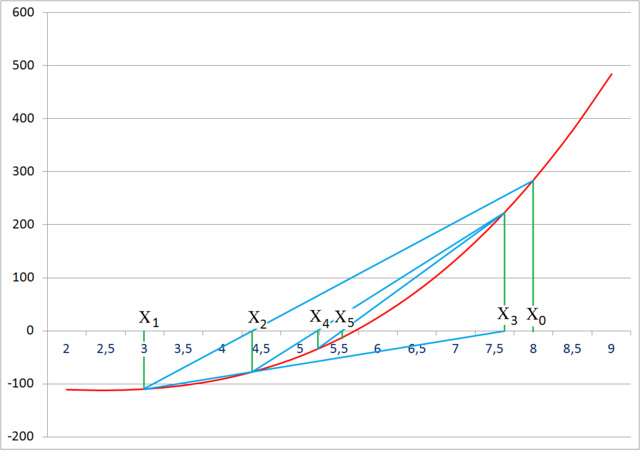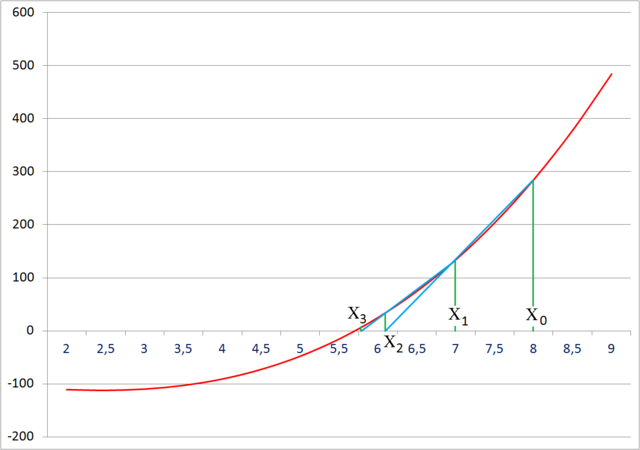homechevron_rightStudychevron_rightMathchevron_rightAlgebra

# Secant method

The secant method is a root-finding algorithm that uses a succession of roots of secant lines to better approximate a root of a function f.This content is licensed under Creative Commons Attribution/Share-Alike License 3.0 (Unported). That means you may freely redistribute or modify this content under the same license conditions and must attribute the original author by placing a hyperlink from your site to this work https://planetcalc.com/3707/. Also, please do not modify any references to the original work (if any) contained in this content.

A brief secant method description can be found below the calculator#### Secant method

Digits after the decimal point: 4
Formula

The file is very large. Browser slowdown may occur during loading and creation.
x

### Secant method

The secant method can be thought of as a finite difference approximation of Newton's method, where a derivative is replaced by a secant line.

We use the root of a secant line (the value of x such that y=0) as a root approximation for function f.

Suppose we have starting values x0 and x1, with function values f(x0) and f(x1).
The secant line has the equation

Hence, the root of secant line (where у=0) is

This is a recurrence relation for the secant method. A graphical interpretation can be seen below.Source

The secant method does not require that the root remains bracketed, like the bisection method does (see below), and hence it does not always converge.Source

As can be seen from the recurrence relation, the secant method requires two initial values, x0 and x1, which should ideally be chosen to lie close to the root.

The tolerance condition can be either:

— function value is less than ε.

— the difference between two subsequent хk is less than ε.

More: Secant method

URL copied to clipboard